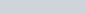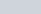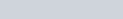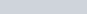# Time transformation

The length part of the Galilean transformation is:with the relative velocity v. The time part of the Galilean transformation is:so that time is the same for all observers.

Einstein made time relative and symmetric with length (at least in one dimension) by assuming an absolute speed of light, c. With β = v/c and γ = 1 − 1/(1 − β²), the Lorentz transformation isA linear transformation would be simpler, i.e., a rigid or Euclidean transformation of time, with a dual one for length. Let w = 1/v, thenAn illustration of this transformation is how time on the Earth is defined by the relative position of the Sun. Locally at noon the Sun is directly overhead, etc. This has been standardized with time zones, although the physical background is continuous. In this case w = 1 hour per 15 degrees of longitude or 4 minutes per degree. With distance measured by degrees of longitude, this is a rigid transformation.